## On a planet where g = 10.0 m/s2 and air resistance is negligible, a sled is at rest on a rough inclined hill rising at 30°. The initial posi

Question

On a planet where g = 10.0 m/s2 and air resistance is negligible, a sled is at rest on a rough inclined hill rising at 30°. The initial position is 8.0 m up the hill along the slope from the bottom. The object is allowed to move and it stops on a rough horizontal surface, at a distance of 4.0 m from the bottom of the hill. The coefficient of kinetic friction on the hill is 0.40. What is the coefficient of kinetic friction between the horizontal surface and the sled?

in progress 0
2 weeks 2021-08-30T18:59:59+00:00 2 Answers 0 views 0

Coefficient of kinetic friction = 0.31

Explanation:

g = 10 m/s²

distance covered up the hill, S = 8 m

Horizontal distance covered, d = 4 m

From the free body diagram attached: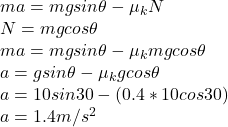The velocity at the bottom can be calculated by: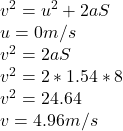Work done through to frictional force = change in kinetic energy: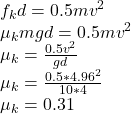Explanation:

a is the acceleration

μ is the coefficient of friction

Acceleration of the object is given by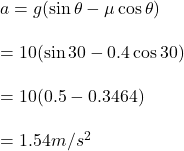Velocity at the bottom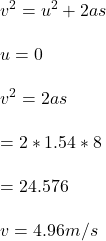after travelling 4m , its velocity becomes 0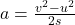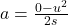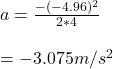Coefficient of kinetic friction

μ = F/N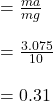Therefore, the Coefficient of kinetic friction is 0.31